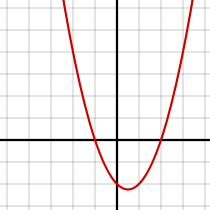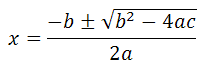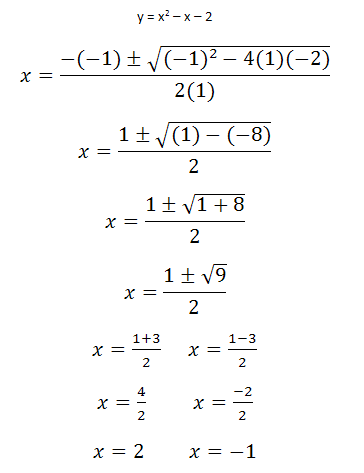# FUN with FUNctions! – Quadratic FunctionsOkay, so we tricked you into clicking this link by telling you it would be fun, but functions are important for both the SAT and the ACT, so sit tight and read through; anyway, we’ve made this explanation as painless as possible.

A quadratic function is a kind of polynomial function. The standard form of a quadratic function is:

y = ax2 + bx + c, where a ≠ 0

A polynomial function is a function that can be defined by evaluating a polynomial; in the case of a quadratic function, “polynomial” simply refers to the exponent. Evaluating a polynomial (in this case) basically means what the function will look like on a graph. The term quadratic means squared, or to the power of two.

What you need to know for the SAT & the ACT: a quadratic function will always result in a parabola; a parabola is a U-shaped figure that will open upwards or downwards. Look at this picture of a quadratic function:You will also have to know that, for all functions in which y = ax2 + bx + c, where a ≠ 0, c will indicate the y-intercept. So, using the above illustration as an example, we know that the quadratic function of this parabola will look like this:

y = ax2 + bx + (-2), or

y = ax2 + bx – 2

We know this because the graph intercepts the y axis at (-2).  (Note: the value of a will determine whether the parabola faces up or down. If a is positive, the parabola will face upwards; if a is negative, the parabola will face downwards)

You will also need to be able to identify the x-intercepts of a quadratic function, and use them to graph the function. You can do this by factoring. Consider this example:

y = x2 – x – 2

y = (x + 1)(x -2)

x = -1, x = 2

(Note: If you are taking the ACT, you will have to be familiar with the quadratic formula; if you are only taking the SAT, then you will only have to be able to factor) If you are unfamiliar with factoring, too lazy to factor or the problem just looks too difficult to factor, you can use the quadratic formula:The quadratic formula lets you solve for x using a, b, and c. Using the same quadratic function above, we can solve for the x intercepts without factoring (keep in mind, where there is no number in front of x, you can always multiply by one):